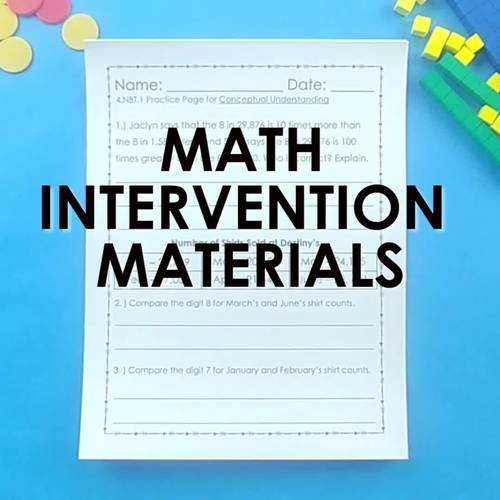# 3rd Grade Math Intervention Pack Algebraic Thinking 3.OA RTI NO PREP Guided MathSubject
Resource Type
File Type

PDF

(8 MB|130 pages)
Standards
Also included in:
1. Purchase this COMPLETE BUNDLE and SAVE!!This bundle includes FIVE math intervention sets (Sets that cover all the Numbers and Operations in Base Ten Standards, Operations and Algebraic Thinking Standards, Fraction Standards, Measurement & Data, and Geometry Standards).You will also receive a gra
\$33.96
\$26.00
Save \$7.96
• Product Description
• StandardsNEW

This resource pack is everything you need to assess and provide intervention for struggling 3rd grade students in the domain: Operations and Algebraic Thinking.

How do these intervention packs work?

Starting with a pretest and item analysis of each question on the test, you will be able to pin-point exact needs of all students. From there printables and short assessments are provided for each standard that assess procedural and conceptual understanding. Data charts and documents are provided to help keep you organized and focused during all steps of the intervention process.

Take the guess work out of providing intervention and focus on what is really important… helping your students!

~~~~~~~~~~~~~~~~~~~~~~~~~~~~~~~~~~~~~~~~~~~~~~~~~~~~

Standards & Topics Covered

Operations & Algebraic Thinking

➥ 3.OA.1 - Interpreting products of whole numbers

➥ 3.OA.2 – Interpreting quotients of whole numbers

➥ 3.OA.3 – Use multiplication and division to solve word problems

➥ 3.OA.4 – Determining unknown numbers in a multiplication or division equation

➥ 3.OA.5 – Apply properties of operations to multiply and divide

➥ 3.OA.6 – Understand division as an unknown-factor problem.

➥ 3.OA.7 – Fluently multiply and divide within 100

➥ 3.OA.8 – Solve two-step word problems using the four operations

➥ 3.OA.9 – Understanding patterns on a multiplication chart

~~~~~~~~~~~~~~~~~~~~~~~~~~~~~~~~~~~~~~~~~~~~~~~~~~~~

What is procedural understanding?

✓ Houses practice of procedural steps

✓ Requires facts, drills, algorithms, methods, etc.

✓ Based on memorizing steps

✓ Students are learning how to do something

***************************************************************************

What is conceptual understanding?

✓ Understanding key concepts and apply prior knowledge to the new concepts

✓ Understanding why something is done

✓ Making connections & relationships

Identify arithmetic patterns (including patterns in the addition table or multiplication table), and explain them using properties of operations. For example, observe that 4 times a number is always even, and explain why 4 times a number can be decomposed into two equal addends.
Solve two-step word problems using the four operations. Represent these problems using equations with a letter standing for the unknown quantity. Assess the reasonableness of answers using mental computation and estimation strategies including rounding.
Fluently multiply and divide within 100, using strategies such as the relationship between multiplication and division (e.g., knowing that 8 × 5 = 40, one knows 40 ÷ 5 = 8) or properties of operations. By the end of Grade 3, know from memory all products of two one-digit numbers.
Understand division as an unknown-factor problem. For example, find 32 ÷ 8 by finding the number that makes 32 when multiplied by 8.
Apply properties of operations as strategies to multiply and divide. Examples: If 6 × 4 = 24 is known, then 4 × 6 = 24 is also known. (Commutative property of multiplication.) 3 × 5 × 2 can be found by 3 × 5 = 15, then 15 × 2 = 30, or by 5 × 2 = 10, then 3 × 10 = 30. (Associative property of multiplication.) Knowing that 8 × 5 = 40 and 8 × 2 = 16, one can find 8 × 7 as 8 × (5 + 2) = (8 × 5) + (8 × 2) = 40 + 16 = 56. (Distributive property.)
Total Pages
130 pages
Included
Teaching Duration
1 month
Report this Resource to TpT
Reported resources will be reviewed by our team. Report this resource to let us know if this resource violates TpT’s content guidelines.